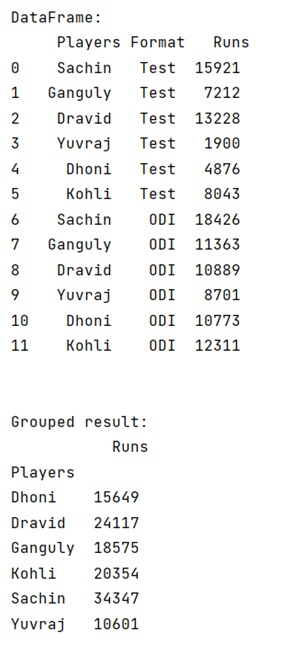# How to perform pandas groupby() and sum()?

Learn how to perform pandas groupby() and sum()?
Submitted by Pranit Sharma, on May 07, 2022

Pandas is a special tool that allows us to perform complex manipulations of data effectively and efficiently. Inside pandas, we mostly deal with a dataset in the form of DataFrame. DataFrames are 2-dimensional data structures in pandas. DataFrames consist of rows, columns, and the data. DataFrame can be created with the help of python dictionaries or lists but in the real world, CSV files are imported and then converted into DataFrames.

## pandas.DataFrame.groupby() Method

On the other hand, groupby() is a simple but very useful concept in pandas. By using groupby(), we can create a grouping of certain values and perform some operations on those values.

The groupby() function split the object, apply some operations, and then combines them to create a group, hence large amount of data and computations can be performed on these groups.

One of the simplest methods on groupby objects is the sum() method.

Syntax:

```DataFrame.groupby(
by=None,
axis=0,
level=None,
as_index=True,
sort=True,
group_keys=True,
squeeze=NoDefault.no_default,
observed=False,
dropna=True
)
```

To work with pandas, we need to import pandas package first, below is the syntax:

```import pandas as pd
```

Let us understand with the help of an example:

```# Importing pandas package
import pandas as pd

# creating a dictionary of cricketers
d = {
"Players":['Sachin','Ganguly','Dravid','Yuvraj','Dhoni','Kohli',
'Sachin','Ganguly','Dravid','Yuvraj','Dhoni','Kohli'],
"Format":['Test','Test','Test','Test','Test','Test',
'ODI','ODI','ODI','ODI','ODI','ODI'],
"Runs":[15921,7212,13228,1900,4876,8043,
18426,11363,10889,8701,10773,12311]
}

# Now we will create DataFrame
df = pd.DataFrame(d)

# Viewing the DataFrame
print("DataFrame:\n",df,"\n\n")

# Performing sum on groupby on Players with runs
result = df.groupby(['Players']).sum()

# Display Result
print("Grouped result:\n",result)
```

Output:Languages: » C » C++ » C++ STL » Java » Data Structure » C#.Net » Android » Kotlin » SQL
Web Technologies: » PHP » Python » JavaScript » CSS » Ajax » Node.js » Web programming/HTML
Solved programs: » C » C++ » DS » Java » C#
Aptitude que. & ans.: » C » C++ » Java » DBMS
Interview que. & ans.: » C » Embedded C » Java » SEO » HR
CS Subjects: » CS Basics » O.S. » Networks » DBMS » Embedded Systems » Cloud Computing
» Machine learning » CS Organizations » Linux » DOS
More: » Articles » Puzzles » News/Updates